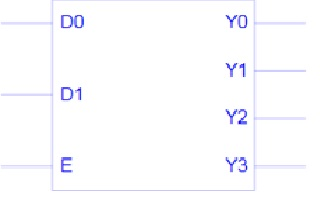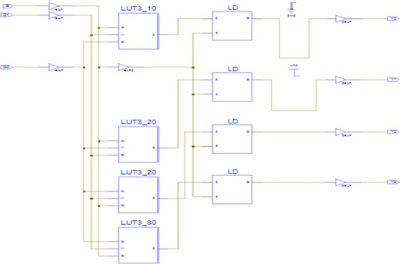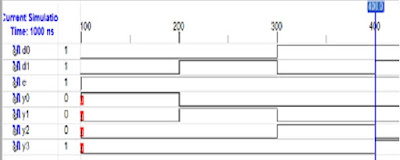## VHDL code for 2 to 4 De-multiplexerVHDL

Object- Write a program (WAP) in VHDL Design, simulate & synthesis of 2:4 De-multiplexer using if-else statement and finally implement it on FPGA board.

Software required: Xilinx ISE 9.2i, PC, Power supply.

VHDL Code:

library IEEE;
use IEEE.STD_LOGIC_1164.ALL; use IEEE.STD_LOGIC_ARITH.ALL;
use IEEE.STD_LOGIC_UNSIGNED.ALL;
---- Uncomment the following library declaration if instantiating
---- any Xilinx primitives in this code.
--library UNISIM;
--use UNISIM.VComponents.all; entity dec_24 is
Port ( D0,D1,E : in STD_LOGIC; Y0,Y1,Y2,Y3 : out STD_LOGIC);
end dec_24;
architecture Behavioral of dec_24 is begin
PROCESS(D0,D1,E) BEGIN
IF(D0='0' AND D1='0' AND E='1')
THEN Y0<='1';
Y1<='0';
Y2<='0';
Y3<='0';
ELSIF(D0='0' AND D1='1' AND E='1')
THEN Y0<='0';
Y1<='1';
Y2<='0';
Y3<='0';
ELSIF(D0='1' AND D1='0' AND E='1') THEN Y0<='0';
Y1<='0';
Y2<='1';
Y3<='0';
ELSIF(D0='1' AND D1='1' AND E='1') THEN Y0<='0';
Y1<='0';
Y2<='0';
Y3<='1';
END IF;
END PROCESS;

end Behavioral;

# Block diagramBlock Diagram

Logical DiagramLogical Diagram

Technical view:Technical View

Truth table

 E D1 D0 Y3 Y2 Y1 Y0 1 0 0 0 0 0 1 1 0 1 0 0 1 0 1 1 0 0 1 0 0 1 1 1 1 0 0 0

Waveforms:Waveforms

Result: I have simulated 2:4 de-mux using if-else statement &                      verified it.